# Multiplication and Division problems

#### Number of problems found: 122

• Quotient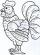Determine the quotient (q) and the remainder (r) from division numbers 100 and 8. Take the test of correctness.
• Division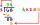Divide by the number 0.2 is the same as multiply by what number?
• What isWhat is the value of the smaller of a pair of numbers for which their sum is 78 and their division quotients are 0.3?
• EvaluateThe division of numbers 18 and 6 increase by product of the numbers 156 and 0.001
• Numbers divisionWith what number should be divided mixed number 2 3/4 to get 11/12?
• DivisionDivision has 18 members: 10 girls and 6 boys, 2 leaders. How many different patrols can be created, if one patrol is 2 boys, 3 girls and 1 leader?
• Two divided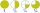Two divided by nine tenths.
• MilimetersHow many millimeters is 1/4 meters?
• NumberCalculate the integer number which, divided by 34 gives 10 and the rest 25.
• Promile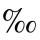Calculate the 4.6 ‰ of 199.How much and how many times is 72.1 greater than 0.00721?
• Divisors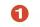Find all divisors of number 493. How many are them?
• Women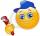In an company of 1050 employees are 2/3 women. How many women work in the company?
• Products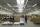15 products are 48 € more expensive than 12 products. How many euros will it cost five same products?
• Ratio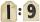Unfold number 1963 in the ratio 9:2:2.
• Chocolates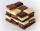In the market we have 3 kinds of chocolates. How many ways can we buy 14 chocolates?
• In the bowlThere are 12 pears in the bowl. Erik ate a third. How many pears remained in the bowl?
• Wallpaper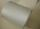Salesman account for 2.3 meters of wallpaper 149.50 Kc. How much cost 1 meter of wallpaper?
• No. of divisorsHow many different divisors have number ??
• Number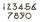What number I think? The third is 6 and half is 2 more than the its quarter.

Do you have an interesting mathematical word problem that you can't solve it? Submit a math problem, and we can try to solve it.

We will send a solution to your e-mail address. Solved examples are also published here. Please enter the e-mail correctly and check whether you don't have a full mailbox.

Please do not submit problems from current active competitions such as Mathematical Olympiad, correspondence seminars etc...